# Digital Circuits - Conversion of Flip-Flops

In previous chapter, we discussed the four flip-flops, namely SR flip-flop, D flip-flop, JK flip-flop & T flip-flop. We can convert one flip-flop into the remaining three flip-flops by including some additional logic. So, there will be total of twelve flip-flop conversions.

Follow these steps for converting one flip-flop to the other.

• Consider the characteristic table of desired flip-flop.

• Fill the excitation values (inputs) of given flip-flop for each combination of present state and next state. The excitation table for all flip-flops is shown below.

Present State Next State SR flip-flop inputs D flip-flop input JK flip-flop inputs T flip-flop input
Q(t) Q(t+1) S R D J K T
0 0 0 x 0 0 x 0
0 1 1 0 1 1 x 1
1 0 0 1 0 x 1 1
1 1 x 0 1 x 0 0
• Get the simplified expressions for each excitation input. If necessary, use Kmaps for simplifying.

• Draw the circuit diagram of desired flip-flop according to the simplified expressions using given flip-flop and necessary logic gates.

Now, let us convert few flip-flops into other. Follow the same process for remaining flipflop conversions.

## SR Flip-Flop to other Flip-Flop Conversions

Following are the three possible conversions of SR flip-flop to other flip-flops.

• SR flip-flop to D flip-flop
• SR flip-flop to JK flip-flop
• SR flip-flop to T flip-flop

### SR flip-flop to D flip-flop conversion

Here, the given flip-flop is SR flip-flop and the desired flip-flop is D flip-flop. Therefore, consider the following characteristic table of D flip-flop.

D flip-flop input Present State Next State
D Q(t) Q(t + 1)
0 0 0
0 1 0
1 0 1
1 1 1

We know that SR flip-flop has two inputs S & R. So, write down the excitation values of SR flip-flop for each combination of present state and next state values. The following table shows the characteristic table of D flip-flop along with the excitation inputs of SR flip-flop.

D flip-flop input Present State Next State SR flip-flop inputs
D Q(t) Q(t + 1) S R
0 0 0 0 x
0 1 0 0 1
1 0 1 1 0
1 1 1 x 0

From the above table, we can write the Boolean functions for each input as below.

$$S=m_{2}+d_{3}$$

$$R=m_{1}+d_{0}$$

We can use 2 variable K-Maps for getting simplified expressions for these inputs. The k-Maps for S & R are shown below.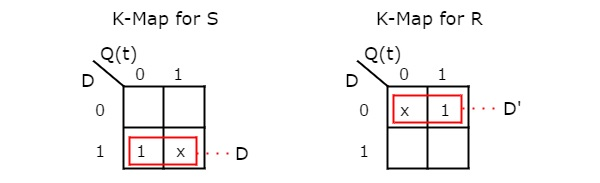So, we got S = D & R = D' after simplifying. The circuit diagram of D flip-flop is shown in the following figure.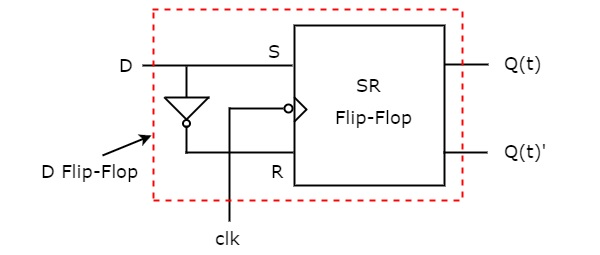This circuit consists of SR flip-flop and an inverter. This inverter produces an output, which is complement of input, D. So, the overall circuit has single input, D and two outputs Q(t) & Q(t)'. Hence, it is a D flip-flop. Similarly, you can do other two conversions.

## D Flip-Flop to other Flip-Flop Conversions

Following are the three possible conversions of D flip-flop to other flip-flops.

• D flip-flop to T flip-flop
• D flip-flop to SR flip-flop
• D flip-flop to JK flip-flop

### D flip-flop to T flip-flop conversion

Here, the given flip-flop is D flip-flop and the desired flip-flop is T flip-flop. Therefore, consider the following characteristic table of T flip-flop.

T flip-flop input Present State Next State
T Q(t) Q(t + 1)
0 0 0
0 1 1
1 0 1
1 1 0

We know that D flip-flop has single input D. So, write down the excitation values of D flip-flop for each combination of present state and next state values. The following table shows the characteristic table of T flip-flop along with the excitation input of D flip-flop.

T flip-flop input Present State Next State D flip-flop input
T Q(t) Q(t + 1) D
0 0 0 0
0 1 1 1
1 0 1 1
1 1 0 0

From the above table, we can directly write the Boolean function of D as below.

$$D=T\oplus Q\left ( t \right )$$

So, we require a two input Exclusive-OR gate along with D flip-flop. The circuit diagram of T flip-flop is shown in the following figure.This circuit consists of D flip-flop and an Exclusive-OR gate. This Exclusive-OR gate produces an output, which is Ex-OR of T and Q(t). So, the overall circuit has single input, T and two outputs Q(t) & Q(t)’. Hence, it is a T flip-flop. Similarly, you can do other two conversions.

## JK Flip-Flop to other Flip-Flop Conversions

Following are the three possible conversions of JK flip-flop to other flip-flops.

• JK flip-flop to T flip-flop
• JK flip-flop to D flip-flop
• JK flip-flop to SR flip-flop

### JK flip-flop to T flip-flop conversion

Here, the given flip-flop is JK flip-flop and the desired flip-flop is T flip-flop. Therefore, consider the following characteristic table of T flip-flop.

T flip-flop input Present State Next State
T Q(t) Q(t + 1)
0 0 0
0 1 1
1 0 1
1 1 0

We know that JK flip-flop has two inputs J & K. So, write down the excitation values of JK flip-flop for each combination of present state and next state values. The following table shows the characteristic table of T flip-flop along with the excitation inputs of JK flipflop.

T flip-flop input Present State Next State JK flip-flop inputs
T Q(t) Q(t + 1) J K
0 0 0 0 x
0 1 1 x 0
1 0 1 1 x
1 1 0 x 1

From the above table, we can write the Boolean functions for each input as below.

$$J=m_{2}+d_{1}+d_{3}$$

$$K=m_{3}+d_{0}+d_{2}$$

We can use 2 variable K-Maps for getting simplified expressions for these two inputs. The k-Maps for J & K are shown below.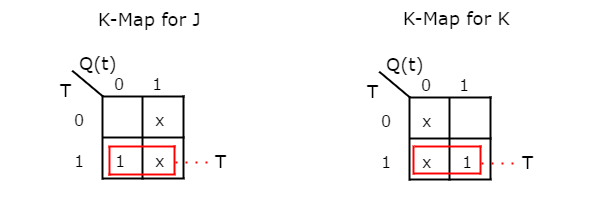So, we got, J = T & K = T after simplifying. The circuit diagram of T flip-flop is shown in the following figure.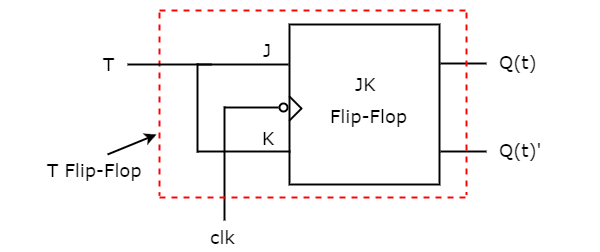This circuit consists of JK flip-flop only. It doesn’t require any other gates. Just connect the same input T to both J & K. So, the overall circuit has single input, T and two outputs Q(t) & Q(t)’. Hence, it is a T flip-flop. Similarly, you can do other two conversions.

## T Flip-Flop to other Flip-Flop Conversions

Following are the three possible conversions of T flip-flop to other flip-flops.

• T flip-flop to D flip-flop
• T flip-flop to SR flip-flop
• T flip-flop to JK flip-flop

### T flip-flop to D flip-flop conversion

Here, the given flip-flop is T flip-flop and the desired flip-flop is D flip-flop. Therefore, consider the characteristic table of D flip-flop and write down the excitation values of T flip-flop for each combination of present state and next state values. The following table shows the characteristic table of D flip-flop along with the excitation input of T flip-flop.

D flip-flop input Present State Next State T flip-flop input
D Q(t) Q(t + 1) T
0 0 0 0
0 1 0 1
1 0 1 1
1 1 1 0

From the above table, we can directly write the Boolean function of T as below.

$$T=D\oplus Q\left ( t \right )$$

So, we require a two input Exclusive-OR gate along with T flip-flop. The circuit diagram of D flip-flop is shown in the following figure.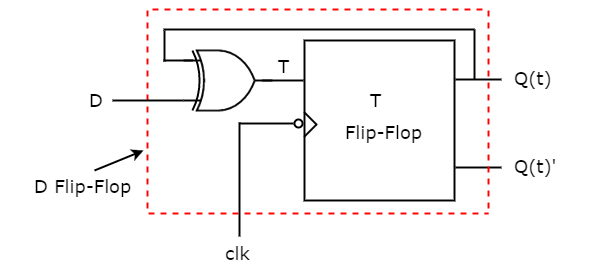This circuit consists of T flip-flop and an Exclusive-OR gate. This Exclusive-OR gate produces an output, which is Ex-OR of D and Q(t). So, the overall circuit has single input, D and two outputs Q(t) & Q(t)’. Hence, it is a D flip-flop. Similarly, you can do other two conversions.